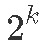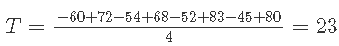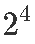# 假设调查人员有兴趣检查减肥干预方法的三个组成部分。

• 记录食物日记（是/否）
• 增加活动（是/否）
• 家访（是/否）

• 要执行因子设计，您需要为多个因子（变量）中的每一个选择固定数量的水平，然后以所有可能的组合运行实验。
• 这些因素可以是定量的或定性的。
• 定量变量的两个水平可以是两个不同的温度或两个不同的浓度。
• 定性因素可能是两种类型的催化剂或某些实体的存在和不存在。

# 测试：

• 有多少因素？
• 每个因素有多少个水平？
• 多少实验条件（运行）？

(a) 有 2+2+1 = 5 个因数。

(b) 两个因素有4个水平，2个因素有3个水平，1个因素有2个水平。

(c) 有 288 个实验条件。

# R语言因子实验设计nlme拟合非线性混合模型分析有机农业施氮水平

## 执行因子设计

• 为每个因子选择固定数量的水平。
• 以所有可能的组合运行实验。

## 立方图

• 立方体展示了这种设计如何沿着立方体的 12 个边缘进行 12 次比较：温度变化影响的四个测量值；浓度变化影响的四种测量方法；催化剂变化效应的四种测量方法。
• 在立方体的每条边上，只有一个因子发生变化，而其他两个因子保持不变。
``````bh4 <- lm
Plot``````

T 的主要（平均）影响是

C的主要（平均）影响是

K 的主要影响是

K 的主要（平均）影响是

# 三因素相互作用

## 结果解释

``qt(p = 1-.025,df = 8)``

T 的 95% 置信区间为

K 的 95% 置信区间为

``1.5-3.2 #下限``
``## \[1\] -1.7``
``1.5+3.2 #上限``
``## \[1\] 4.7``

## 交互图

``plot(tabT,taC,ty, type = "l")``

## 2k 因子设计的线性模型

yi 是第 i 次运行的结果，

2^3 因子设计的线性模型是：

``````fact.mod <-lm(y~T\*K\*C,data = tab0503)
round(summary(fact.mod)\$coefficients,2)``````

``model.matr``

•• 4 的除数将对比度转换为两个平均值之间的差异。
• 通过乘以各自因素的标识获得交互作用对比。
• 每列相对于其他列完全平衡（正数和负数相等）。
• 平衡（正交）设计确保每个估计的效应不受其他效应的大小的影响。

``````fad <-lm
round(2*coeffits,2)``````

``2*confint.lm``

## 非重复因子设计中的正态图

### 回顾正态分位数图

N(0,1)的累积分布函数 (CDF) 具有 S 形。

``````x <- seq
plot(x,pnorm)``````

``````N <- 1000
x <- rnorm(N)
p <- ((1:N)-0.5)/N
plot``````

``plot(qnorm(p),sort(x))``

``qqnorm(x);qqline(x)``

1. 正态假设不成立。
2. 方差不是恒定的。

• 当一些效应不为零时，相应的估计效应将趋于更大并偏离直线。
• 对于正面影响，估计的影响落在该线之上，而负面影响落在该线之下。

# 示例 –研究化学反应的设计

``fct1 <- lm``

``Plot(fac``

## Lenth 方法：检验没有方差估计的实验的显着性

``ef <- 2*fat1\$coeffic``

s0=1.5⋅median∣∣^θi∣∣的估计是

``````s0 <- 1.5*median(abs(eff))
s0``````

``2.5*s0``

∣∣^θi∣∣≥2.5s0 的效应 ^θi 将被修剪。下面是标记为 `TRUE` ( `x1,x2,x4,x2:x4`)的效应

``abs(eff)<2.5*s0``

``````PE <- 1.5*median
PE``````

ME 和 SME 是

``````ME <- PE*qt
ME``````
``PE*qt(p =(1+.95^{1/15})/2,df=(16-1)/3)``

``````lor <- round(ef-ME,2)
uper <- round(ef+ME,2)
kable(cbind)``````

``Plot(fat1,cex.fac = 0.5)``

Kaizong Ye拓端研究室（TRL）的研究员。

​非常感谢您阅读本文，如需帮助请联系我们！QQ在线咨询

15121130882

0571-63341498

## 关注有关新文章的微信公众号

This will close in 0 seconds